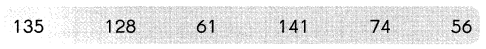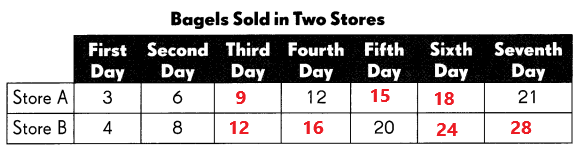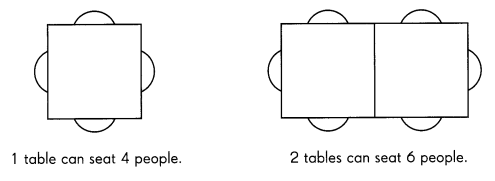# Math in Focus Grade 4 Chapter 2 Answer Key Estimation and Number Theory

Go through the Math in Focus Grade 4 Workbook Answer Key Chapter 2 Estimation and Number Theory to finish your assignments.

## Math in Focus Grade 4 Chapter 2 Answer Key Estimation and Number Theory

Challenging Practice

Question 1.
The estimated difference between two numbers is 60. Find two numbers that when rounded to the nearest ten, have a difference of 60. Use the numbers in the box.After rounding the numbers to the nearest ten, they will be as below.
140        130       60        140       70      60
By seeing the above numbers and to get an estimated difference between any two numbers is 60, the possible combinations will be 130 and 70 (130 – 70 = 60).
So, the two numbers and 130 and 70.

Question 2.
When a 3-digit number is divided by a 1 -digit number, the estimated quotient is 50. Think of two possible numbers that can give this quotient. Then check if your answer is correct.
The three digits numbers are 100, 150.
The one digit numbers are 2, 5.
When a 3-digit number is divided by a 1 -digit number, the estimated quotient is 50.
100/2 = 50
150 /3 = 50

Question 3.
A given number is a multiple of 4. It is between 6 and 1 5. It is a factor of 16. What is the number?
The numbers which are multiples of 4 and between 6 and 15 are 8 and 12.
As it also should be a factor of 16, The required number should be 8.

Question 4.
When a 3-digit number is rounded to the nearest ten and to the nearest hundred, the answer is the same. What is one possible number that fits this rule?
The one possible number that fits the rule is 199.
If 199 rounded to the nearest tens its 200 and rounded to the nearest hundred its 200.

Question 5.
The number of bagels sold each day in two stores follows a pattern. Complete the table below to show this pattern.Fill in the blanks using the data from the table above.

a. How many bagels did Store B sell on the seventh day? ____
Store B sold 28 bagels on the seventh day.

b. The two stores sold the same number of bagels on different days. Which were the days?
Store A: ____ Store B: __
Store A sold 12 bagels on fourth day.
Store B sold 12 bagels on third day.

Problem Solving

Question 1.
Mr. Chan bought some pencils for a group of students. If he gives them 2 pencils each, he will have 10 pencils left. If he gives them 3 pencils each, he will have none left. How many students are in the group?There are 10 students in the group.
If he give 2 pencils each, that’s 20 pencils, but he has 10 left.
2 x 10 = 20
30 – 20 = 10
If he give 3 pencils each, that’s 30. No pencils left over.
3 x 10 = 30
30 – 30 = 0

Question 2.
On the opening day at a toy store, every third customer gets a ball and every fourth customer gets a stuffed animal. Sixty people come to the store. How many get both a ball and a stuffed animal?